# Chapter 2 Boolean Algebra and Digital Logic

 Page 1/4 Date 10.09.2018 Size 339.25 Kb. #63066
1   2   3   4

Digital Technology and Computer Fundamentals

Chapter 2

Boolean Algebra and Digital Logic

## Exercise

2.1. Refer to Example 2.0, if the Sales Manager is also authorized to sign a cheque and the conditions for validity of the cheque remain unchanged, find the truth table for the validity of a cheque.

2.2. Find the truth table of the following expressions:

i. F =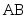+ (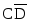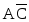)

ii. F =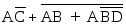iii. F =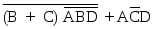2.3. Draw the logic circuit diagrams for the functions in exercise 2.0.

2.4. Given the logic circuit in Figure 2.0, find the logical expression of function F.

2.5. Prove by truth table the followings:

i.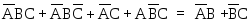ii.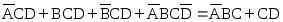iii.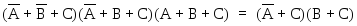iv.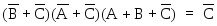v.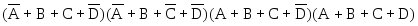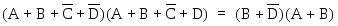vi.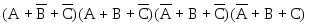=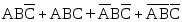vii.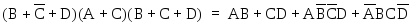viii. (A +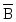+ C) (A ++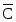) (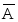+ B + C) (++ C)

=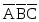+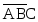+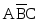+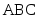2.6. Prove by Boolean Algebraic theorems the expressions in exercise 2.0.

2.7. From the following truth tables, find the expressions for the functions in both standard sum of products and standard product of sums forms.

i.
 Inputs Functions A B C P Q 0 0 0 0 1 0 0 1 0 1 0 1 0 0 0 0 1 1 1 0 1 0 0 0 1 1 0 1 1 1 1 1 0 0 0 1 1 1 1 1

ii.
 Inputs Functions A B C D X Y Z 0 0 0 0 0 1 0 0 0 0 1 1 0 1 0 0 1 0 0 0 1 0 0 1 1 0 0 0 0 1 0 0 0 1 0 0 1 0 1 1 0 1 0 1 1 0 0 1 1 0 1 1 1 0 0 0 1 0 0 0 1 1 0 1 0 0 1 0 1 0 1 0 1 0 1 0 0 1 0 1 1 0 1 0 1 1 0 0 1 0 1 1 1 0 1 0 0 0 1 1 1 0 0 1 1 1 1 1 1 0 0 1

2.8. Find the simplified expressions in both sum of products and product of sums forms using Boolean Algebraic theorems for the functions P, Q, X, Y, and Z in exercise 2.0.

2.9. Simplify the following expressions to sum of products form using Boolean Algebraic theorems:

i.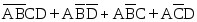ii.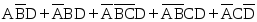iii.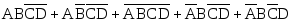2.10. Simplify the following expressions to product of sums from using Boolean Algebraic theorems:

i.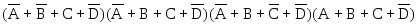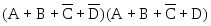ii.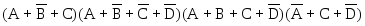iii.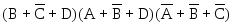2.11. Use Karnaugh-map to find the simplified expressions of functions P, Q, X, Y, and Z, in exercise 2.0, in both sum of products and product of sums form. Draw the logic circuits for the simplified functions.

2.12. Simplify the following expressions to sum of products form using Karnaugh-map method. Synthesis the logic circuit for the functions with NAND gates only.

i. F(A, B, C) = S (0, 2, 3, 6)

ii. F(A, B, C, D) = S (1, 3, 7, 12, 13,14, 15)

iii. F(A, B, C, D) = S (2, 3, 4, 6, 7,11, 15)

iv. F(A, B, C, D) = S (6, 7, 9, 11, 13, 14, 15)

2.13. Simplify the following expressions to product of sums form using Karnaugh-map method. Synthesis the logic circuit for the functions with NOR gates only.

i. F(A, B, C) = P (1, 2, 3, 5)

ii. F(A, B, C, D) = P (0, 1, 3, 4, 7, 8, 12, 15)

iii. F(A, B, C, D) = P (0, 2, 4, 8, 10, 12, 14)

iv. F(A, B, C, D) = P (1, 4, 5, 10, 12, 13, 14)

2.14. Use combinational circuit to solve the problem in exercise 2.0.

2.15. The English subject in a school consists of four parts: Essay, Comprehension, Grammar and Oral. A student passes the English subject if the student

i. passes all four parts; or

ii. passes Oral and any other two parts; or

iii. passes Essay and any other two parts; or

iv. passes Essay and Oral.

Use four variables (A, B, C, D) to represent the four parts of the English subject respectively, i.e. A represents Essay and a logic 1 represents pass in Essay while a logic 0 represents fail in Essay. Find the function, P, in terms of the four variables, that represents whether a student passes the subject. Use a combinational circuit to solve the problem.